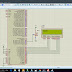Today I am going to write about ADC (Analogue to Digital Converter). ADC coverts the analogue values, eg- a sensor's value, to digital form. The value depends on the voltage drop in the sensor.

LPC2148 have
two 10 bit ADCs. It means that the value can vary from 0 to 1023.
Measurement range from 0 to 3v.
Global start command for both conversions.

ADC is used by most of the sensors for eg- temperature sensor, force sensor, IR sensor, potentiometer, and many more. All these sensors send the data to the microcontroller in analogue form, which then converts it into digital values.

Registers Used:

AD0CR:- A/D Control Register.
1.) Bits[7-0]: SEL- Selects which of the AD0.7:0/AD1.7:0 pins is (are) to be sampled and converted. For AD0, bit 0 selects Pin AD0.0, and bit 7 selects pin AD0.7.
2.) Bits[15-8]: CLKDIV- The VPB clock (PCLK) is divided by (this value plus one) to produce the clock for the A/D converter, which should be less than or equal to 4.5 MHz.
3.) Bit: BURST- Set this bit to 1 for doing repeated conversions, and set it to 0 for software controlled conversions.
4.) Bits[19-17]: CLKS- This field selects the number of clocks used for each conversion in Burst mode, and the number of bits of accuracy of the result in the RESULT bits of ADDR, between 11 clocks (10 bits) and 4 clocks (3 bits).
000 11 clocks / 10 bits
001 10 clocks / 9bits
010 9 clocks / 8 bits
011 8 clocks / 7 bits
100 7 clocks / 6 bits
101 6 clocks / 5 bits
110 5 clocks / 4 bits
111 4 clocks / 3 bits
5.) Bit: PDN-         1 The A/D converter is operational.
0 The A/D converter is in power-down mode.
6.) Bits[26-24]: START-
When the BURST bit is 0, these bits control whether and when an A/D conversion is started:
000 No start (this value should be used when clearing PDN to 0).
001 Start conversion now.
010 Start conversion when the edge selected by bit 27 occurs on P0.16/EINT0/MAT0.2/CAP0.2 pin.
011 Start conversion when the edge selected by bit 27 occurs on P0.22/TD3/CAP0.0/MAT0.0 pin.
100 Start conversion when the edge selected by bit 27 occurs on MAT0.1.
101 Start conversion when the edge selected by bit 27 occurs on MAT0.3.
110 Start conversion when the edge selected by bit 27 occurs on MAT1.0.
111 Start conversion when the edge selected by bit 27 occurs on MAT1.1.

AD0GDR:- A/D Global Data Register. It contains the ADC's DONE bit and the result of the most recent conversion.
1.) Bits[15-6]: BRESULT- When DONE is 1, this contains a binary fraction representing the voltage on Ain pin selected by the SEL field, divided by the voltage on Vref pin, V/Vref.
2.) Bit: DONE- This bit is set to 1 when an A/D conversion completes. It is cleared when this register is used.

Some Insight into the Code:-

AD0CR|=(14<<8) -->  shifts the 14 to the 8th bit. Here the conversion clock will be 60/(14+1) = 4 MHz as mentioned above.
AD0CR|= (1<<21) --> Set PDN to 1 thus A/D is operational.
AD0CR|=(1<<24) --> start conversion

CODE:-

#include <LPC214X.H>

#include "pll.h"
#include "lcd.h"
#include <stdlib.h>
#include <stdint.h>

int main ()

{
uint32_t data;
char chdata;
PINSEL1|=(1<<24);
PINSEL0=0;
PINSEL2=0;
IODIR0=0x0fffffff;   // set Port0 as output
lcd_init ();    // initialise LCD
pll_init();     // initialise clocks
VPBDIV=0x01;    // setting Pclk at 60 MHz
while (1)
{
AD0CR=(1<<1)|(14<<8)|(1<<21); // select AD0.1, 4 MHz clock, PDN=1
while (!(AD0GDR&(1<<31)));   // wait untill DONE bit is set
data=((AD0GDR>>6)&0x3ff);   // get the data from register
sprintf (chdata, "%04u", data);    // convert the data
decode_cmd (0x80);   // set the cursor to Row 1 ,Col 1
send_string (chdata);    // display the data
}

}

This is it guys, you can check the working in the attached file below. ADC is important if you want to use sensors, as I mentioned we mostly use A/D conversion to get the values from most of the sensors.

Hope you guys understood the concept here.

Subscribe for more tutorials. Please hit +1 and check out my youtube channel for programming the real hardware.
ADC in LPC2148Reviewed by arun singh on July 01, 2017 Rating: 5